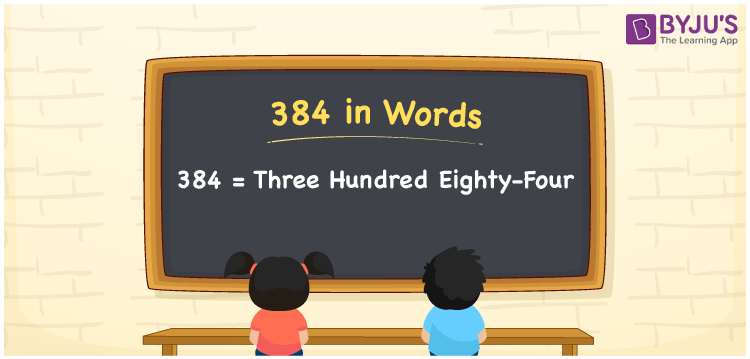# 384 in Words

384 in words is Three hundred eighty-four. We can easily convert the number 384 into words with the help of a place value chart. Also, Three hundred eighty-four is the word form or number name of 384. The number name is used to express or spell the number. For example, Rs. 384 can be spelt as Three hundred eighty-four rupees. Let’s understand how to convert the cardinal number 384 into word form here in this article.

 384 in words Three hundred eighty-four Three hundred eighty-four in Numbers 384

## 384 in English Words

Generally, we express numbers in words using the English alphabet. Thus, we spell 384 in English words as “Three hundred eighty-four”.## How to Write 384 in Words?

The number 384 contains four digits so let’s create a place value chart with four columns to convert the number 384 to word form. This can be done as follows.

 Hundreds Tens Ones 3 8 4

Here, ones = 4, tens = 8, hundreds = 3

These numbers can be written as:

3 × Hundred + 8 × Ten + 4 × One

= 3 × 100 + 8 × 10 + 4 × 1

= 300 + 80 + 4

= Three hundred + Eighty + Four

= Three hundred eighty-four

Therefore, 384 in words = Three hundred eighty-four

As we know, 384 is a natural number that precedes 385 and succeeds 383.

384 in words – Three hundred eighty-four

Is 384 an odd number? – No

Is 384 an even number? – Yes

Is 384 a prime number? – No

Is 384 a composite number? – Yes

Is 384 a perfect square number? – No

Is 384 a perfect cube number? – No

## Frequently Asked Questions on 384 in Words

Q1

### How do you write 384 in word form?

We can write the number 384 in word form as Three hundred eighty-four.
Q2

### How do you write Three hundred eighty-four as numerals?

We can write the numeral for Three hundred eighty-four as 384.
Q3

### How to write Rs. 384 in words on a cheque?

On a cheque, we generally write an amount of Rs. 384 in words as “Three hundred eighty-four rupees only”.